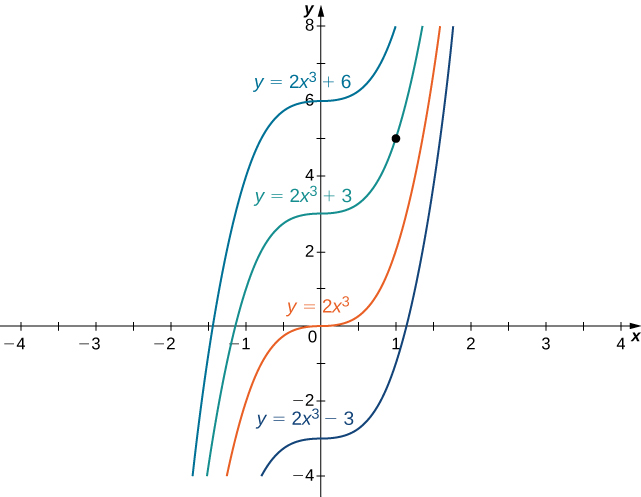# 4.10 Antiderivatives  (Page 4/10)

 Page 4 / 10

## Evaluating indefinite integrals

Evaluate each of the following indefinite integrals:

1. $\int \left(5{x}^{3}-7{x}^{2}+3x+4\right)dx$
2. $\int \frac{{x}^{2}+4\sqrt{x}}{x}dx$
3. $\int \frac{4}{1+{x}^{2}}dx$
4. $\int \text{tan}\phantom{\rule{0.1em}{0ex}}x\phantom{\rule{0.1em}{0ex}}\text{cos}\phantom{\rule{0.1em}{0ex}}x\phantom{\rule{0.1em}{0ex}}dx$
1. Using [link] , we can integrate each of the four terms in the integrand separately. We obtain
$\int \left(5{x}^{3}-7{x}^{2}+3x+4\right)dx=\int 5{x}^{3}dx-\int 7{x}^{2}dx+\int 3x\phantom{\rule{0.1em}{0ex}}dx+\int 4dx.$

From the second part of [link] , each coefficient can be written in front of the integral sign, which gives
$\int 5{x}^{3}dx-\int 7{x}^{2}dx+\int 3x\phantom{\rule{0.1em}{0ex}}dx+\int 4dx=5\int {x}^{3}dx-7\int {x}^{2}dx+3\int x\phantom{\rule{0.1em}{0ex}}dx+4\int 1dx.$
Using the power rule for integrals, we conclude that
$\int \left(5{x}^{3}-7{x}^{2}+3x+4\right)dx=\frac{5}{4}{x}^{4}-\frac{7}{3}{x}^{3}+\frac{3}{2}{x}^{2}+4x+C.$
2. Rewrite the integrand as
$\frac{{x}^{2}+4\sqrt{x}}{x}=\frac{{x}^{2}}{x}+\frac{4\sqrt{x}}{x}=0.$

Then, to evaluate the integral, integrate each of these terms separately. Using the power rule, we have
$\begin{array}{cc}\hfill \int \left(x+\frac{4}{{x}^{2\text{/}3}}\right)dx& =\int x\phantom{\rule{0.1em}{0ex}}dx+4\int {x}^{-2\text{/}3}dx\hfill \\ & =\frac{1}{2}{x}^{2}+4\frac{1}{\left(\frac{-2}{3}\right)+1}{x}^{\left(-2\text{/}3\right)+1}+C\hfill \\ & =\frac{1}{2}{x}^{2}+12{x}^{1\text{/}3}+C.\hfill \end{array}$
3. Using [link] , write the integral as
$4\int \frac{1}{1+{x}^{2}}dx.$

Then, use the fact that ${\text{tan}}^{-1}\left(x\right)$ is an antiderivative of $\frac{1}{\left(1+{x}^{2}\right)}$ to conclude that
$\int \frac{4}{1+{x}^{2}}dx=4\phantom{\rule{0.1em}{0ex}}{\text{tan}}^{-1}\left(x\right)+C.$
4. Rewrite the integrand as
$\text{tan}\phantom{\rule{0.1em}{0ex}}x\phantom{\rule{0.1em}{0ex}}\text{cos}\phantom{\rule{0.1em}{0ex}}x=\frac{\text{sin}\phantom{\rule{0.1em}{0ex}}x}{\text{cos}\phantom{\rule{0.1em}{0ex}}x}\phantom{\rule{0.1em}{0ex}}\text{cos}\phantom{\rule{0.1em}{0ex}}x=\text{sin}\phantom{\rule{0.1em}{0ex}}x.$

Therefore,
$\int \text{tan}\phantom{\rule{0.1em}{0ex}}x\phantom{\rule{0.1em}{0ex}}\text{cos}\phantom{\rule{0.1em}{0ex}}x=\int \text{sin}\phantom{\rule{0.1em}{0ex}}x=\text{−}\text{cos}\phantom{\rule{0.1em}{0ex}}x+C.$

Evaluate $\int \left(4{x}^{3}-5{x}^{2}+x-7\right)dx.$

${x}^{4}-\frac{5}{3}{x}^{3}+\frac{1}{2}{x}^{2}-7x+C$

## Initial-value problems

We look at techniques for integrating a large variety of functions involving products, quotients, and compositions later in the text. Here we turn to one common use for antiderivatives that arises often in many applications: solving differential equations.

A differential equation is an equation that relates an unknown function and one or more of its derivatives. The equation

$\frac{dy}{dx}=f\left(x\right)$

is a simple example of a differential equation. Solving this equation means finding a function $y$ with a derivative $f.$ Therefore, the solutions of [link] are the antiderivatives of $f.$ If $F$ is one antiderivative of $f,$ every function of the form $y=F\left(x\right)+C$ is a solution of that differential equation. For example, the solutions of

$\frac{dy}{dx}=6{x}^{2}$

are given by

$y=\int 6{x}^{2}dx=2{x}^{3}+C.$

Sometimes we are interested in determining whether a particular solution curve passes through a certain point $\left({x}_{0},{y}_{0}\right)$ —that is, $y\left({x}_{0}\right)={y}_{0}.$ The problem of finding a function $y$ that satisfies a differential equation

$\frac{dy}{dx}=f\left(x\right)$

$y\left({x}_{0}\right)={y}_{0}$

is an example of an initial-value problem . The condition $y\left({x}_{0}\right)={y}_{0}$ is known as an initial condition . For example, looking for a function $y$ that satisfies the differential equation

$\frac{dy}{dx}=6{x}^{2}$

and the initial condition

$y\left(1\right)=5$

is an example of an initial-value problem. Since the solutions of the differential equation are $y=2{x}^{3}+C,$ to find a function $y$ that also satisfies the initial condition, we need to find $C$ such that $y\left(1\right)=2{\left(1\right)}^{3}+C=5.$ From this equation, we see that $C=3,$ and we conclude that $y=2{x}^{3}+3$ is the solution of this initial-value problem as shown in the following graph.Some of the solution curves of the differential equation d y d x = 6 x 2 are displayed. The function y = 2 x 3 + 3 satisfies the differential equation and the initial condition y ( 1 ) = 5 .

## Solving an initial-value problem

Solve the initial-value problem

$\frac{dy}{dx}=\text{sin}\phantom{\rule{0.1em}{0ex}}x,y\left(0\right)=5.$

First we need to solve the differential equation. If $\frac{dy}{dx}=\text{sin}\phantom{\rule{0.1em}{0ex}}x,$ then

$y=\int \text{sin}\left(x\right)dx=\text{−}\text{cos}\phantom{\rule{0.1em}{0ex}}x+C.$

Next we need to look for a solution $y$ that satisfies the initial condition. The initial condition $y\left(0\right)=5$ means we need a constant $C$ such that $\text{−}\text{cos}\phantom{\rule{0.1em}{0ex}}x+C=5.$ Therefore,

$C=5+\text{cos}\left(0\right)=6.$

The solution of the initial-value problem is $y=\text{−}\text{cos}\phantom{\rule{0.1em}{0ex}}x+6.$

any genius online ? I need help!!
Pina
need to learn polynomial
Zakariya
i will teach...
nandu
I'm waiting
Zakariya
evaluate the following computation (x³-8/x-2)
teach me how to solve the first law of calculus.
what is differentiation
f(x) = x-2 g(x) = 3x + 5 fog(x)? f(x)/g(x)
fog(x)= f(g(x)) = x-2 = 3x+5-2 = 3x+3 f(x)/g(x)= x-2/3x+5
diron
pweding paturo nsa calculus?
jimmy
how to use fundamental theorem to solve exponential
find the bounded area of the parabola y^2=4x and y=16x
what is absolute value means?
Chicken nuggets
Hugh
🐔
MM
🐔🦃 nuggets
MM
(mathematics) For a complex number a+bi, the principal square root of the sum of the squares of its real and imaginary parts, √a2+b2 . Denoted by | |. The absolute value |x| of a real number x is √x2 , which is equal to x if x is non-negative, and −x if x is negative.
Ismael
find integration of loge x
find the volume of a solid about the y-axis, x=0, x=1, y=0, y=7+x^3
how does this work
Can calculus give the answers as same as other methods give in basic classes while solving the numericals?
log tan (x/4+x/2)
Rohan
Rohan
y=(x^2 + 3x).(eipix)
Claudia
Ismael
A Function F(X)=Sinx+cosx is odd or even?
neither
David
Neither
Lovuyiso
f(x)=1/1+x^2 |=[-3,1]
apa itu?
fauzi
determine the area of the region enclosed by x²+y=1,2x-y+4=0
Hi
MP
Hi too
Vic
hello please anyone with calculus PDF should share
Which kind of pdf do you want bro?
Aftab
hi
Abdul
can I get calculus in pdf
Abdul
explain for me
Usman
okay I have such documents
Fitzgerald
Hamza

#### Get Jobilize Job Search Mobile App in your pocket Now!By Robert MurphyBy OpenStaxBy Anh DaoBy Sandhills MLTBy RhodesBy Jazzycazz JacksonBy Jessica CollettBy Stephen VoronBy OpenStaxBy Madison Christian